Question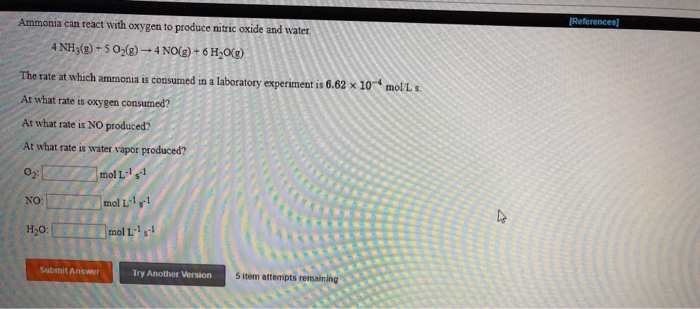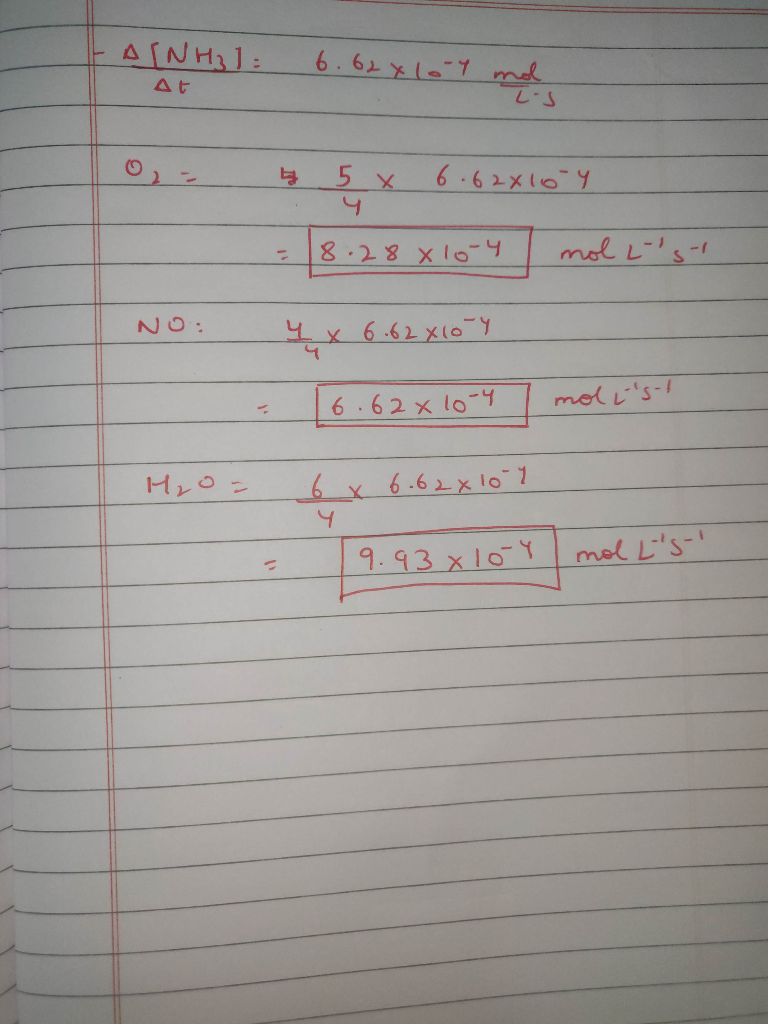#### Earn Coins

Coins can be redeemed for fabulous gifts.

Similar Homework Help Questions
• ### Ammonia reacts with oxygen to form nitric oxide and water vapor: 4NH3 +5O2 → 4NO + 6H2O

Ammonia reacts with oxygen to form nitric oxide and water vapor: 4NH3 +5O2 → 4NO + 6H2O What is the theoretical yield of water, in moles, when 40.0g NH3 and 50.0g O2 are mixed and allowed to react: 1.87 mol 3.53 mol 1.57 mol 1.30 mol None of these

• ### Ammonia reacts with diatomic oxygen to form nitric oxide and water vapor:

Always show your work. No credit will be given for any answers (even if they are correct) for which work is not shown. Ammonia reacts with diatomic oxygen to form nitric oxide and water vapor: 4NH3 + 502 ---> 4NO + 6H2O What is the theoretical yield of water, in moles, when 40.0 g NH3 and 50.0g, O2 are mixed and allowed to react?

• ### Ammonia (NH3) chemically reacts with oxygen gas (O2) to produce nitric oxide (NO) and water (H2O)...

Ammonia (NH3) chemically reacts with oxygen gas (O2) to produce nitric oxide (NO) and water (H2O) . What mass of oxygen gas is consumed by the reaction of 8.8g of ammonia? Be sure your answer has the correct number of significant digits

• ### Ammonia NH3 chemically reacts with oxygen gas O2 to produce nitric oxide NO and water H2O...

Ammonia NH3 chemically reacts with oxygen gas O2 to produce nitric oxide NO and water H2O . What mass of oxygen gas is consumed by the reaction of 7.02g of ammonia? Be sure your answer has the correct number of significant digits.

• ### Ammonia reacts with oxygen to form nitric oxide and water vapor: 4NH3 + 5O2 ---> 4NO...

Ammonia reacts with oxygen to form nitric oxide and water vapor: 4NH3 + 5O2 ---> 4NO + 6H2O When 20.0 g NH3 and 50.0 g O2 are allowed to react, which is the limiting reagent? A) NO B) O2 C) H2O D) no reagent is limiting E) NH3 What is the coefficient for O2 when the following combustion reaction of a hydrocarbon is balanced? ___C7H14 + ___ O2 ---> ___ CO2 + __ H2O A) none of these B) 10...

• ### 6.62. With a platinum catalyst, ammonia will burn in oxygen to give nitric oxide, NO. tions...6.62. With a platinum catalyst, ammonia will burn in oxygen to give nitric oxide, NO. tions 4NH, () + 50(9) + 4NO(g) + 6H2O(): AN ---906 kJ uccess Center What is the enthalpy change for the following reaction? NO () - HO (9) + NH3 (a) + (9) wered by Quizlet

• ### References) The reaction for the Haber process, the industrial production of ammonia, NG) + 3H2(g) +...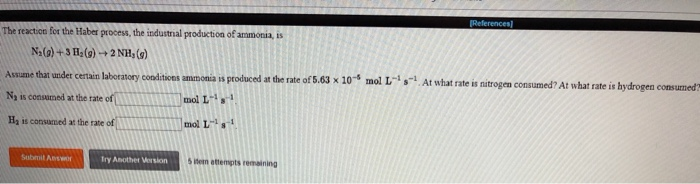References) The reaction for the Haber process, the industrial production of ammonia, NG) + 3H2(g) + 2NH,() Awsume that under certain laboratory conditions ammonia is produced at the rate of 5.63 x 10" mol L s At what rate is nitrogen consumed? At whatrate is hydrogen consumed! N, is consumed at the rate of 111111111111 mol L Hy is consed at the rate of 11 mol L- Try Another Version item attempts remaining

• ### 1) Ammonia, NH3, reacts with molecular oxygen, O2, to formnitric oxide, NO, and water:4NH3(g)...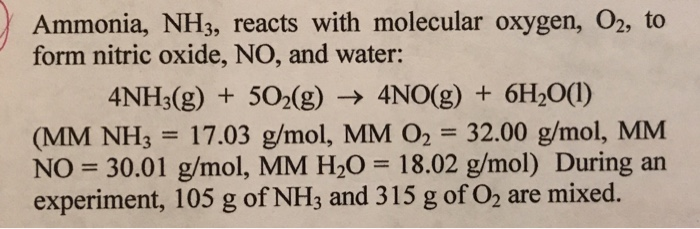1) Ammonia, NH3, reacts with molecular oxygen, O2, to form nitric oxide, NO, and water:4NH3(g) + 5O2(g) = 4NO (g) +6H2O(l)A. What is the limiting reactant and what is the theoretical yield of NO?B. What is the theoretical yield of H2O?C. How many grams of excess reagent will be left over?D. If the actual yield of NO had been 91 g, what would be the percent yield of the reaction

• ### Nitric oxide (NO) can be produced by partially oxidizing ammonia (NH3) with oxygen via the following...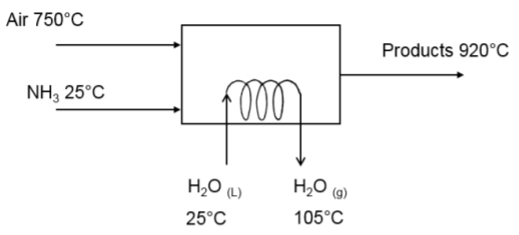Nitric oxide (NO) can be produced by partially oxidizing ammonia (NH3) with oxygen via the following reaction: 2NH3 + 2.5O2 = 2NO + 3H2O (1) Ammonia can also react with oxygen via the following reactions: 2NH3 + 1.5O2 = N2 + 3H2O (2) 2NH3 + 3.5O2 = 2NO2 + 3H2O (3) Ammonia is fed to a reactor at 25 °C and 500 kg/h. Dry air (21 mol % O2, 79 mol % N2) enters at 8000 kg/h. Eighty percent (80%)...

• ### Ammonia (NH,) chemically reacts with oxygen gas (02) to produce nitric oxide (NO) and water (H,0)...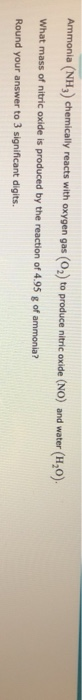Ammonia (NH,) chemically reacts with oxygen gas (02) to produce nitric oxide (NO) and water (H,0) What mass of nitric oxide is produced by the reaction of 4.95 g of ammonia? Round your answer to 3 significant digits.